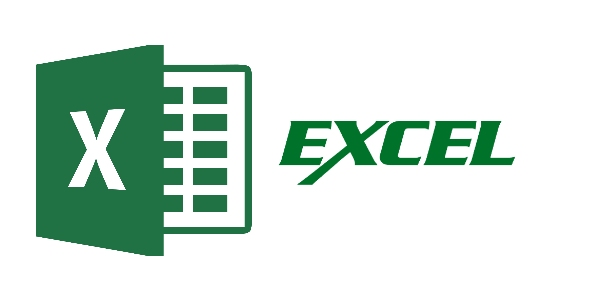# Excel 2010 (Level 3 - Advanced)

10 Questions | Attempts: 1115Settings• 1.
How many conditions can the sumif check for?
• A.

1

• B.

2

• C.

3

• D.

4

• 2.
By default how many worksheets are in a workbook?
• A.

1

• B.

2

• C.

3

• D.

4

• 3.
An nested if statement only allows for one argument?
• A.

True

• B.

False

• 4.
What is a pivot table?
• A.

A report

• B.

• C.

A filter

• D.

Vlookup

• 5.
A macro is a part of a conditional format?
• A.

True

• B.

False

• 6.
If the range lookup is false in a vlookup what does it mean?
• A.

The result will return an exact match

• B.

The result will return the nearest match

• C.

It will return a negative

• D.

None of the above

• 7.
A template is a worksheet which has all the necessary features you require?
• A.

True

• B.

False

• 8.
What is a scenario?
• A.

Figures which switch between varying amounts

• B.

A conditional format

• C.

A macro

• D.

An IF statement

• 9.
The sumif function has the...... range, criteria, sum_range?
• A.

True

• B.

False

• 10.
A lookup table counts the columns in the Col_index_No and not the cell reference?
• A.

True

• B.

False

## Related TopicsBack to top
×

Wait!
Here's an interesting quiz for you.